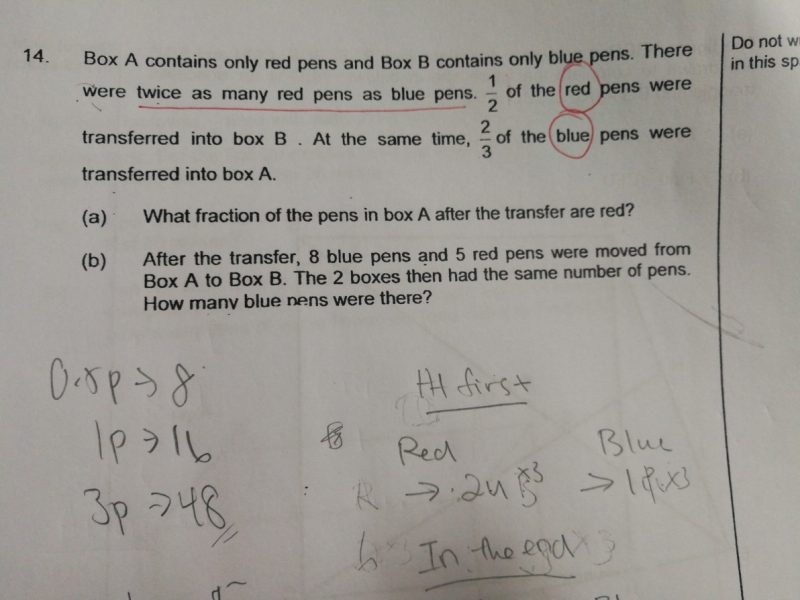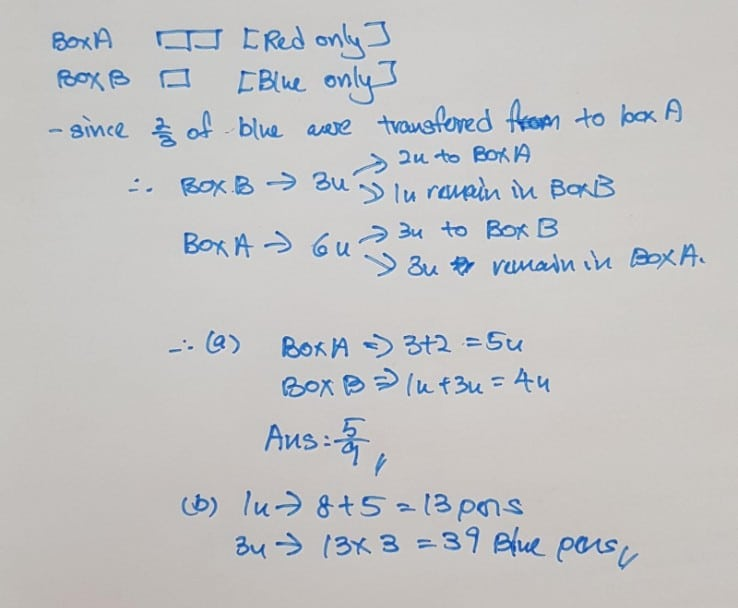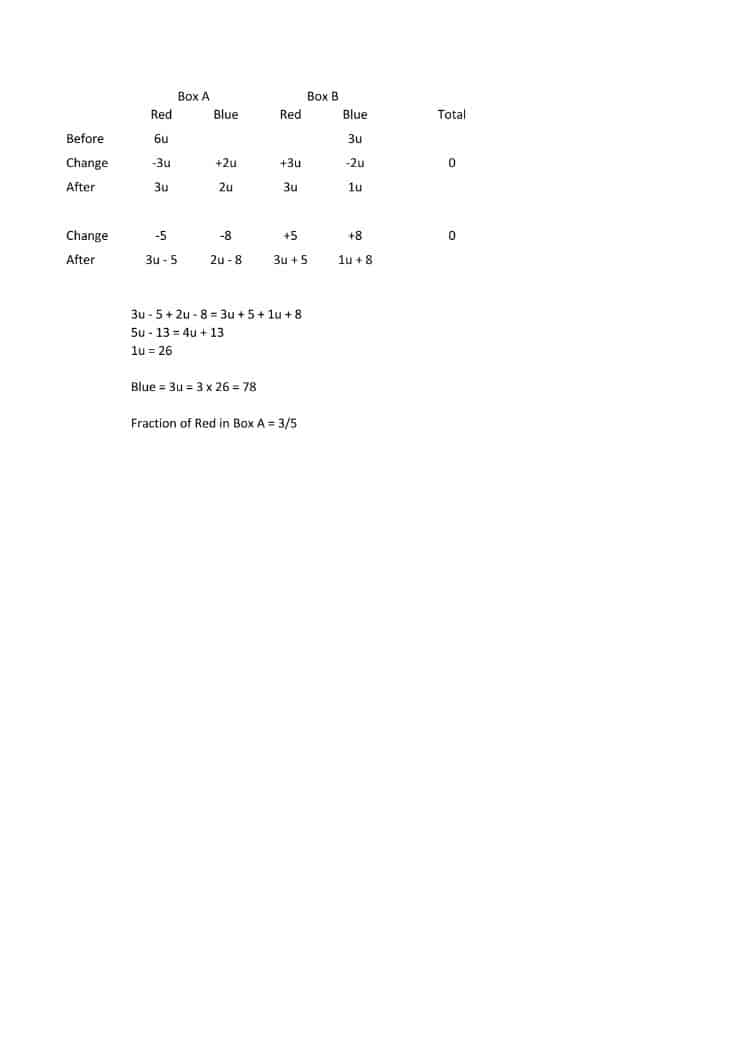# QuestionCan someone help to explain the above question?

Thank you 🤗

(a)
Box A —
red : 6 units – 3 units = 3 units
blue : 2 units
total : 5 units

Box B —
blue : 3 units – 2 units = 1 unit
red : 3 units
total : 4 units

3/(3 + 2) = 3/5

(b)
5 units – 8 – 5 = 4 units + 8 + 5
1 unit = 26
3 units = 78

Ans : (a) 3/5; (b) 78 blue pens.

0 Replies 0 LikesHi

The answer sheet stated for a) 3/5 and b) 78 but yours not telly.

Can explain how did u get this part ?

1 unit ->8+3 ?

Thanks

2 Replies 0 Likes